# Mondrian Conformal Predictor

Mondrian conformal predictors (MCPs) represent a wide class of confidence predictors which is the generalisation of conformal predictors and inductive conformal predictors with a new property of validity, validity within categories; this kind of validity is stronger (for large data sets) than the usual conservative validity for conformal predictors.

## Definition

A Mondrian taxonomy is a measurable function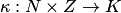, where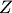is a set of observations and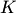is a countable set of elements called categories, that satisfies some natural properties given in Vovk et al. (2005), Section 4.5. The definition involve partitions into rectangles, reminiscent of Piet Mondrian's paintings (see also Scott Locklin's blog). However, in this article we will use a simpler definition given in Balasubramanian et al. (2014), Section 2.2.

A simple Mondrian taxonomy is a functionthat maps any finite sequence of observations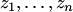to a same-length sequence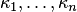of categories that is equivariant with respect to permutations: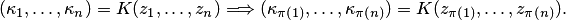We refer to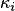as the category of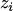. A-conditional conformity measure is a measurable function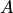that maps every data sequenceto a same-length sequence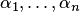and that is equivariant with respect to permutations leaving the categories intact.

The Mondrian conformal predictor determined by a-conditional conformity measureand a set of significance levels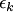,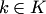, is defined by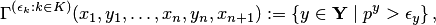where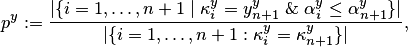where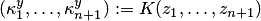and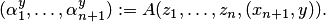(Formally, as defined here, an MCP is a confidence predictor only when allcoincide.)

The standard assumption for MCPs is the randomness assumption (also called the IID assumption).

A simple example is given by label-conditional conformal predictors, which require the label space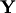to be finite. In this case the set of categories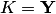coincides with the set of labels, and the category of an observation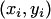is simply its label,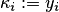.

Another example is where we want to make the analysis conditional on a type of objects. For example, if the objects are people, we might want to define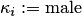if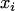is male and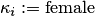ifis female.

## Validity

All the statements in the section are given under the randomness assumption.

Smoothed MCPs are defined analogously to smoothed conformal predictors: the definition of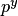is now modified to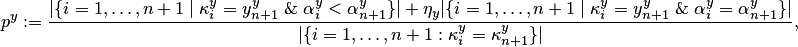and the rest of the definition is the same. Here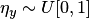are random numbers (chosen independently at different trials and independently of the observations).

In the next theorem we assume thatdepends only on(without this assumption, we can still guarantee the right coverage probability but the independence of errors may be lost).

Theorem Any smoothed MCP is category-wise exact w.r. to, that is, for all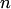, the conditional probability distribution of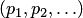given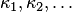is uniform on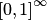, where the observations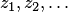are generated from an exchangeable probability distribution on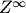and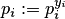are the p-values for the true labels.

This implies that in the long run the frequency of erroneous predictions given by smoothed MCPs on examples of categoryis equal tofor each. Therefore, the frequency of errors made by MCPs on examples of categorydoes not exceedfor each.

An important feature of MCPs is that the theorem only requires the observations in the same category to be exchangeable: it will remain true if the equality in the definition of exchangeability is only required to hold for permutations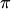leaving the categories intact. A nice example (due to Daniil Ryabko) showing the significance of this relaxation is recognizing handwritten text (assuming the text has been divided into separate symbols): the label-conditional conformal predictor will be valid provided that different instances of the same symbol are exchangeable; we do not need to require that the sequence of symbols in the text be exchangeable (this assumption would be patently wrong).

## Applications

Bibliography

• Vineeth N. Balasubramanian, Shen-Shyang Ho, and Vladimir Vovk, editors (2014). Conformal Prediction for Reliable Machine Learning: Theory, Adaptations, and Applications. Morgan Kaufmann, Chennai.
• Vladimir Vovk, Alexander Gammerman, and Glenn Shafer (2005). Algorithmic learning in a random world. Springer, New York.